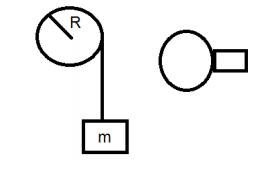# Pulley with a rope

1. Homework Statement
a circular homogenic pulley (disk) with radius R and mass of M hanging on a axis that passes through his center,
the pulley rotating without friction.
we wrap the pulley with a rope with a total mass of mr and length of L and in the other side of the rope we ataching body with a mass of m* , m* is starting to go down while rotating the disk
find the angular velocity of the pullay as function of the length of the suspanded rope -x## Homework Equations

Ipulley=MR^2/2 - inertia torque of the pulley (in the center of mass)
Irope=mr*R^2 - inertia torque of the rope (at the beginning when wrapped)
E=0.5Iω^2 -energy to rotate the disk pulley
U=m*gh-potential energy of the mass m* at the beginning (h is not given)

## The Attempt at a Solution

ive tried to do equations of preserving energy between the start point when we have only potential of the mass m* and to equalize it to the point when we have a total hanging mass of m**= m* + mr(x/L) in a height of (h-x) -potential energy plus kinetic energy of 0.5m**v^2 when v equal to ωR. this energy also going for the rotation of the pulley E=0.5Iω^2. I suceeded by finding the height of h with those equations: h=x+m*L/mr-ω^2R^2/2g-m*ω^2R^2L/2mrg.
but it doesnt helps me finding the relation between x and ω...
I cant find more equation, couldnt think of an equation that link also the inertia of the "ring" of the wrapped rope....

haruspex
Homework Helper
Gold Member
2020 Award
If h is not given, why introduce it? Can't you take it as zero?
By the way, I think your energy equation is not quite right.

If h is not given, why introduce it? Can't you take it as zero?
By the way, I think your energy equation is not quite right.
how could I express potential energy without h? or the potential energy isnt relevantic at all?

haruspex